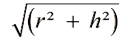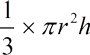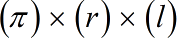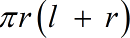Request a Tool

Surface Area of a Cone Calculator

Cone Surface Area calculator use to calculate slant height, curved surface area, total surface area and volume of a cone.

Area
0

Formula• π = a constant value 3.1415
• h = Height of the Cone
• r = Radius of the Cone

Defination / Uses

A cone is a geometric shape with a circle at one end, typically at the base. Every point of a two-dimensional figure is made up of all line segments joining to a single point.

Area
0

Formula• π = a constant value 3.1415
• h = Height of the Cone
• r = Radius of the Cone

Defination / Uses

A cone is a geometric shape with a circle at one end, typically at the base. Every point of a two-dimensional figure is made up of all line segments joining to a single point.

Curved Area
0

Formula• π = a constant value 3.1415
• l = Length of the Cone
• r = Radius of the Cone

Defination / Uses

A cone is a geometric shape with a circle at one end, typically at the base. Every point of a two-dimensional figure is made up of all line segments joining to a single point.

Area
0

Formula• π = a constant value 3.1415
• l = Length of the Cone
• r = Radius of the Cone

Defination / Uses

A cone is a geometric shape with a circle at one end, typically at the base. Every point of a two-dimensional figure is made up of all line segments joining to a single point.

How to use cone area calcultor?

Its a single step procedure.

• Add the height of cube in its given field.

Thats it! You will get your answer in less than a second. Use upper given formulas in case of manual calculation. Any right cone's surface area can be calculated using the surface area of a cone calculator. You'll be incredibly confident about how to find the surface area of a cone after using our calculator and reading this article.

A general cone shape has a circular or oval base with a vertex (or tip) above it that is connected to the base's perimeter. This calculator is for a right cone, which is a specific sort of cone. It has a circular base with a vertex that is directly above the base's centre. As a result, the angle formed by the base and an imaginary line connecting the base and the vertex is 90°, or a right angle. For quick calculation use our weetools. No sign-up, registration OR captcha is required to use this tool.